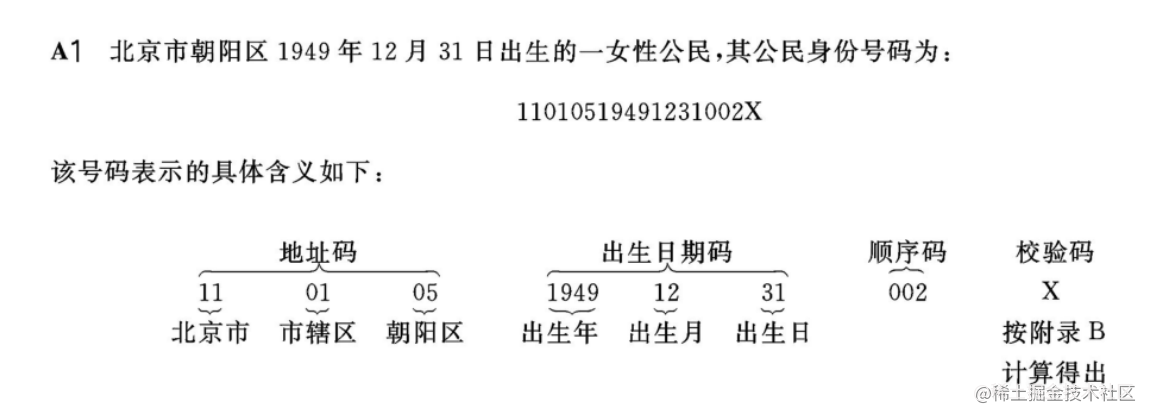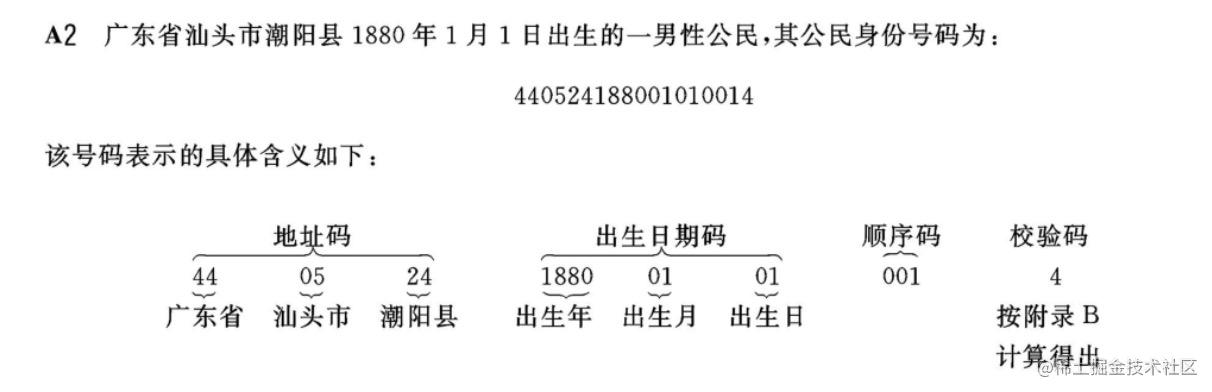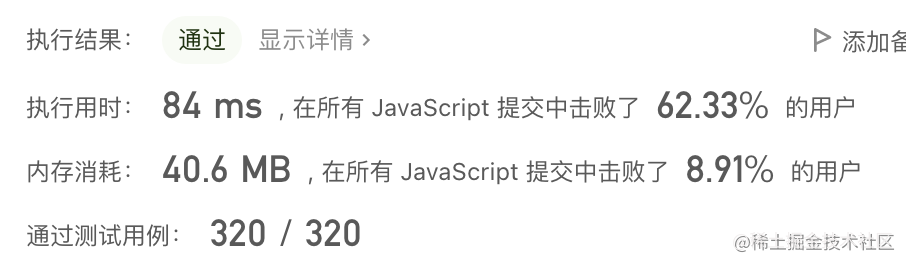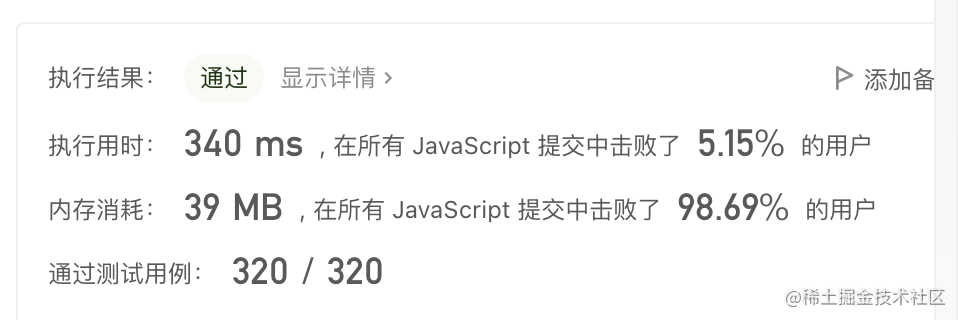# 几个有趣的算法公众号「 微医大前端技术 」## 根据身份证号码计算出性别、年龄

### 一、身份证号码国家标准

#### 1、范围

《公民身份号码》(GB11643-1999)该标准规定了公民身份号码的编码对象、号码的结构和表现形式，使每个编码对象获得一个唯一的、不变的法定号码。 ​

#### 2、号码的结构##### 2.4、校验码

（1）十七位数字本体码加权求和公式：S = Sum(Ai * Wi)

Ai * Wi7905020292427718305004

S = 7 + 9 + 0 + 5 + 0 + 20 + 2 + 9 + 24 + 27 + 7 + 18 + 30 + 5 + 0 + 0 + 4 = 167 ​

（2）计算模：Y = mod(S, 11) Y = 167 % 11 => 2 ​

（3）通过模得到对应的校验码

012345678910

### 二、代码实现

1、身份证号正确性校验

``````const WEIGHT = [7,9,10,5,8,4,2,1,6,3,7,9,10,5,8,4,2]
const MO = [1,0,'X',9,8,7,6,5,4,3,2]

function isRightId(id){
const arr = id.split('')
const checkNumber = arr.pop() // 去除校验码，将 pop 的返回值赋值给 checkNumber
let sum = 0
arr.forEach((ele, index) => {
sum += ele * WEIGHT[index]
})
const m = sum % 11
const result = MO[m]
return result+'' === checkNumber
}

console.log(isRightId('11010519491231002X')) // true
console.log(isRightId('110105194912310029')) // false

2、由身份证号计算年龄

``````function getAge(id){
// 1、先判断身份证号的正确性
// 2、判断是否在世
const year = id.substr(6,4)
const month = id.substr(10,2)
const day = id.substr(12,2)

const timeBrth = new Date(`\${year}/\${month}/\${day}`).getTime()
const timeNow = new Date().getTime()
const longTime = timeNow - timeBrth
const days = longTime / (1*24*60*60*1000)

let result = ''
if(days<31){
result = parseInt(days) + '天'
}else if(days<365){
result = `\${parseInt(days/30)}月\${parseInt(days%30)}天`
}else{
result = `\${parseInt(days/365)}岁\${parseInt(days%365/30)}月\${parseInt(days%365%30)}天`
}
return result
}
console.log(getAge('11010519491231002X')) // 71 岁 8 月 16 天
console.log(getAge('11010520210820002X')) // 6 天
console.log(getAge('11010520210720002X')) // 1 月 7 天

3、由身份证号判断性别

``````function getSex(id){
// 1、先判断身份证号的正确性
const sex = id.substr(16,1)
return sex%2? '男': '女'
}
console.log(getSex('11010519491231002X')) // 女
console.log(getSex('11010520210820001X')) // 男

### 三、其他

1、变性手术后，身份证号码是否更改？

• 跨性别人士身份证性别变更后，依户口所在派出所公示为准，进行身份证号码变更。

2、计算年龄前应先确认是否在世

### 四、参考资料

《公民身份号码》(GB11643-1999)

## 动态规划

### 2、简单：爬楼梯

``````输入： 2

1.  1 阶 + 1 阶
2.  2 阶

``````输入： 3

1.  1 阶 + 1 阶 + 1 阶
2.  1 阶 + 2 阶
3.  2 阶 + 1 阶

``````// 把数据缓存在一个数组中
var climbStairs = function(n) {
let dp = []
dp=1;
dp=1;
for(let i=2;i<=n;i++){
dp[i]=dp[i-1]+dp[i-2];
}
return dp[n];
};

// 使用递归
var climbStairs = function(n) {
if(n===1) return 1
if(n===2) return 2
return climbStairs(n-1) + climbStairs(n-2)
};

f(x)=f(_x_−1)+f(x_−2) 爬到第 x _级台阶的方案数是爬到第 x - 1 级台阶的方案数和爬到第 x - 2 级台阶的方案数的和。 ​

LeetCode 运行结果：### 3、中等：最长回文子串

``````输入：s = "babad"

``````输入：s = "cbbd"

• P(i, i) = true
• P(i, i+1) = (Si == Si+1)

``````    function longestPalindrome (s) {
// 先判断字符串长度，如果为 1 则直接返回
let len = s.length
if (len < 2) return s

// 初始化变量
let maxLen = 1
let begin = 0

// dp[i][j] 表示 s[i..j] 是否是回文串
let dp = []
// 初始化：所有长度为 1 的子串都是回文串
for (let i = 0; i < len; i++) {
dp[i] = []
dp[i][i] = true
}

// 将字符串切割为数组
let charArray = s.split('')

// 递推开始
for (let L = 2; L <= len; L++) { // 枚举子串长度
// 枚举左边界，左边界的上限设置可以宽松一些
for (let i = 0; i < len; i++) {
// 由 L 和 i 可以确定右边界，即 j - i + 1 = L 得
let j = L + i - 1;
// 如果右边界越界，退出当前循环
if (j >= len) {
break;
}
// 判断是否为回文
if (charArray[i] !== charArray[j]) {
dp[i][j] = false
} else {
// 对于一个子串而言，如果它是回文串，并且长度大于 2，那么将它首尾的两个字母去除之后，它仍然是个回文串。
let flag = j - i < 3
dp[i][j] = flag ? true : dp[i + 1][j - 1]
}
// 当 dp[i][L] == true 成立，就表示子串 s[i..L] 是回文，记录回文长度和起始位置
if (dp[i][j] && j - i + 1 > maxLen) {
maxLen = j - i + 1;
begin = i;
}
}
}
return s.substring(begin, begin + maxLen);
}
console.log(longestPalindrome('cbbd'), 'cbbd') // bb cbbd

LeetCode 运行结果：### 4、困难：接雨水

``````输入：height = [0,1,0,2,1,0,1,3,2,1,2,1]

``````输入：height = [4,2,0,3,2,5]

``````function trap(height) {
// 不满足条件，直接返回
let len=height.length;
if(len<=2) return 0;

let maxLeft = []; // 第 i 根柱子左边最高柱子的高度
let maxRight = []; // 第 i 根柱子右边最高柱子的高度

maxLeft = height;
for(let i=1; i<len; i++){
maxLeft[i] = Math.max(height[i], maxLeft[i-1]) // 动态转移
}

maxRight[len-1] = height[len-1];
for(let j=len-2; j>=0; j--){
maxRight[j] = Math.max(height[j], maxRight[j+1]) // 动态转移
}

let sum=0;
for(let i=0;i<len;i++) sum+=Math.min(maxLeft[i],maxRight[i])-height[i];

return sum;
}

LeetCode 运行结果：`````` function trap(height) {
let ans=0;
for(let i=1; i<height.length-1; i++){
let l_hight = height[i];
let r_hight = height[i];

// 找到 i 列右侧最高柱子高度
for(let r=i; r<height.length; r++){
if(height[r]>r_hight) r_hight=height[r];
}

// 找到 i 列柱子左侧最高柱子高度
for(let l=i; l>=0; l--){
if(height[l]>l_hight) l_hight=height[l];
}

ans+=Math.min(l_hight,r_hight)-height[i];
}
return ans;
}

LeetCode 运行结果：### 5、参考资料

leetcode-cn.com/problems/lo…

leetcode-cn.com/problems/tr…

## 贪心算法

### 2、分饼干

``````function findContentChildren1(children, cookies){
children = children.sort((a, b) => a - b)
let childrenLength = children.length
let count = 0
for(let i = 0, j = 0; i < childrenLength && j < cookiesLength; i++, j++){
j++
}
count++
}
}
return count
}

console.log(findContentChildren1([1,2,3], [1,1])) // 1
console.log(findContentChildren1([1,2], [1,2,3])) // 2
console.log(findContentChildren1([1,2,3], [1,1,3,4])) // 3

1. 将孩子的胃口、饼干的大小都按照从小到大排序。
3. 如果满足孩子的胃口，且 j 在范围内，则 count 加 1。

1. 定义函数 findContentChildren，接受 2 个参数，分别为 children：孩子的胃口，cookies 饼干的大小。
2. 将 children 和 cookies 按照从小到大排序。
3. 定义能够满足孩子胃口的个数 count，并最终将 count 返回。
5. 如果 children[i] <= cookies[j]即当前饼干能满足孩子，且 j 在范围内，则 count 加 1。
6. 进入下一次循环（即尝试满足下一个孩子）。

### 3、买股票

``````function maxProfit(list, fee){
const length = list.length
let buy = list + fee // 假定：买入时机为第 1 天
let profit = 0
for(let i = 1; i < length; i++){
}else if(list[i] > buy){ // 如果有利润，则卖出
profit += list[i] - buy // 计算利润
}
}
return profit
}

console.log(maxProfit([1, 3, 2, 8, 4, 9], 2)) // 8
console.log(maxProfit([1,3,7,5,10,3], 3)) // 6

1. 定义函数 maxProfit，接受 2 个参数，list 为股票价格趋势，fee 为手续费
2. 定义 profit，并在最后将 profit 返回
3. 假定：买入时机为第 1 天（即：list）
4. 如果股票价格降低，则调整买入时机为后一天
5. 如果有利润，则卖出，并计算利润，再次调整买入时机（即循环步骤 3、4、5）

1. 假定：买入时机为第 1 天
2. 如果股票价格降低，则调整买入时机为后一天
3. 如果有利润，则卖出，并且计算利润
4. 再次 - 假定：买入时机（循环步骤 1-3）

### 4、情侣牵手

N 对情侣坐在连续排列的 2N 个座位上，想要牵到对方的手。 计算最少交换座位的次数，以便每对情侣可以并肩坐在一起。 一次交换可选择任意两人，让他们站起来交换座位。​ 人和座位用 0 到 2N-1 的整数表示，情侣们按顺序编号，第一对是 (0, 1)，第二对是 (2, 3)，以此类推，最后一对是 (2N-2, 2N-1)。 ​

``````/**
* @param {number[]} row
* @return {number}
*/
var minSwapsCouples = function(row) {
let hashMap = {}; // {人: 位置}
for(let i=0; i<row.length; i++){
hashMap[row[i]] = i
}

let ans = 0; // 交换次数

for(let i=0; i<row.length; i+=2){ // 按照一对遍历
let lover=row[i]^1; // row[i]的情侣
if(hashMap[lover] !== i+1){ // 如果不相邻，就交换
ans++;
hashMap[row[i+1]] = hashMap[lover]; // row[i+1]使用了 lover 的下标
// 交换位置
[row[i+1], row[hashMap[lover]]] = [row[hashMap[lover]], row[i+1]]
hashMap[lover]=i+1; // lover 的下标改为 i+1 使其相邻
}
}
return ans;
};

1. 遍历数组，检查情侣是否相邻，如果不是，就调整一个的位置使其相邻。
2. 我们尽量只挪动情侣中的一位，另一位不动，这样才能保证挪动次数最少。
3. 这样交换，不会影响其他的情侣。

1. 定义函数 minSwapsCouples，接收一个参数 row 即情侣座位关系的数组。
2. 得到{人: 位置}的对象。
3. 定义交换次数 ans，并在最后将其返回。
4. 遍历左侧情侣，检查右侧是否为 TA 的情侣，如果不是，则找到 TA 的情侣并调换座位。

### 5、参考资料